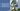# How to calculate First Common Ancestor of two Nodes in Binary Tree

August 14, 2020First try to understand question.

• Its a binary tree, not a binary search tree
• Find first common ancestor
• There is no link to parent nodes

## Initial Thoughts

• First, you can search in the tree, whether these two nodes exists in the tree.
• Proceed only when we found both nodes in the tree
• You can find the location of these two nodes on the tree. There can be three possibilities:
1. If either element is the actual root, then root is the answer
2. Both nodes found on the left OR right side of the root node (same side of root)
3. One is on left, other is on right. Roor is the answer.

For Case-2, on finding that both nodes are on the same side. We will repeat our same algorithm going forward in that side only.

Lets see a code for this.

``````public class CommonAncestor {
public static class Node {
public int data;
public Node left;
public Node right;
public Node(int data) {
this.data = data;
this.left = null;
this.right = null;
}
}

/**
* To check whether a node exists in a root element
*/
private boolean covers(Node root, Node toSearch) {
if (root == null || toSearch == null) {
return false;
}
if (root.data == toSearch.data) {
return true;
}

return this.covers(root.left, toSearch) || this.covers(root.right, toSearch);
}

private Node helper1(Node root, Node node1, Node node2) {
if (root == null) {
return null;
}
if (root.data == node1.data || root.data == node2.data) {
return root;
}

boolean firstNodeFoundOnLeft = this.covers(root.left, node1);
boolean firstNodeFoundOnRight = this.covers(root.left, node2);

if (firstNodeFoundOnLeft != firstNodeFoundOnRight) {
//both are on different sides. Found result.
return root;
}

//both are on the same side.
return this.helper1(firstNodeFoundOnLeft ? root.left : root.right,
node1, node2);
}

public Node findCommonAncestor_1(Node root, Node node1, Node node2) {
if (!this.covers(root, node1) || !this.covers(root, node2)) {
//one or both nodes are not in the tree
System.out.println("Not covered");
return null;
}

return this.helper1(root, node1, node2);
}
}``````

## Code Explanation

• First we check whether our tree covers both the nodes or not. If not, we do not need to go further.
• Then, code starts with `helper1(root, node1, node2)`
• Some conditions, if our root is null. No need to go further. And, if root element becomes equal to any of our search nodes. This is the result.
• We search for both nodes, on the left side of the root node.
• If both are on different sides. Root is the answer
• Else, we will branch on the side (left or right), where both found.

The function calls recursively, and same code repeats.

## Complexity of this code

Consider the tree to be balanced tree.

• Initially `covers()` is called twice. So, its `2 times O(n)`, which computes to `O(n)`
• Our `helper1()` function gets called on branches one time for each node. 2 * n/2 for both nodes. Which comes out to be: `2n/2 + 2n/4 + 2n/8 ~= O(logn)`

Total complexity of this code is `O(n)`